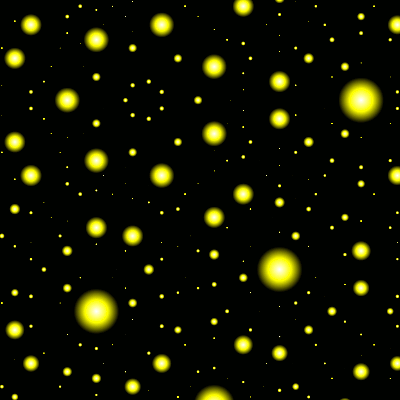This demo requires HTML5 Canvas support.

## Help

What am I seeing here?

• Move: arrow keys and <,, >. or mouse/finger dragging.
• Zoom: + and -, mouse scrolling, or pinch zoom.

Code written by Pteromys and released under the Unlicense.

This page draws quasicrystalline patterns by slicing and projecting higher-dimensional lattices into the plane. Points are sized by their proximity to the slice.

Thanks to the crystallographic restriction theorem, rotational symmetry of order other than 1, 2, 3, 4, or 6 requires a lattice of dimension higher than 2. In fact, to have a 2-D plane that rotates with order $$n$$, the required dimension of the lattice is $$\phi(n)$$. (Why?)

The patterns have some self-similarity—they look the same on high and low magnification. This takes its simplest form when $$n = 5$$; then it has a direct analogue in the 3-D version and is related to the game Lucy and Lily. But when $$n$$ is larger, it gets more complicated.

## Dimension

A rotation preserving a lattice can be written as an integer matrix. If it rotates a 2-D plane with order $$n$$, one of its eigenvalues there is a primitive $$n$$th root of unity $$\zeta_n$$. Then all $$\phi(n)$$ of the other primitive $$n$$th roots of unity—i.e. all of $$\zeta_n$$'s Galois conjugates—are eigenvalues too, so the matrix's size is at least $$\phi(n)$$.

## Zooming

To keep the number of dots drawn roughly constant, as we expand the plane we also uniformly shrink the unseen dimensions. This way as the dots spread out, more dots come in from outside to fill in the gaps.

Self-similarity occurs because there are transformations (realizable as circular shifts in $$n$$ dimensions) taking dots to other dots while scaling the visible two dimensions in place. So the view before and after look the same, yet they differ by some scaling in the visible dimensions!

If $$n = 5, 8, 10,$$ or $$12$$, or in the 3-D version, then this is the full story—exactly as many dimensions are hidden from view as are visible; and such a transformation scales them by reciprocal amounts, exactly undoing some level of zoom.

Taking dots to dots requires that this zoom level is a real unit in the ring of integers of $$\mathbf{Q}(e^{2 \pi i /n})$$, and that the zoom levels in the hidden dimensions are its Galois conjugates, with the same multiplicity. When $$n$$ is not one of the values named above, the visible and hidden dimensions don't match in number; so this kind of transformation can't scale all the hidden dimensions uniformly!

Happily, Dirichlet's unit theorem ensures there are enough units (thus enough transformations) to approximate any level of uniform zooming with bounded error. What results is a coarser sort of self-similarity, where small scales look like large scales if some distortion in the unseen dimensions is acceptable.

Of course, finding a complete set of units is tricky; this program as written only looks for cyclotomic units, which is good enough for $$n < 34$$.